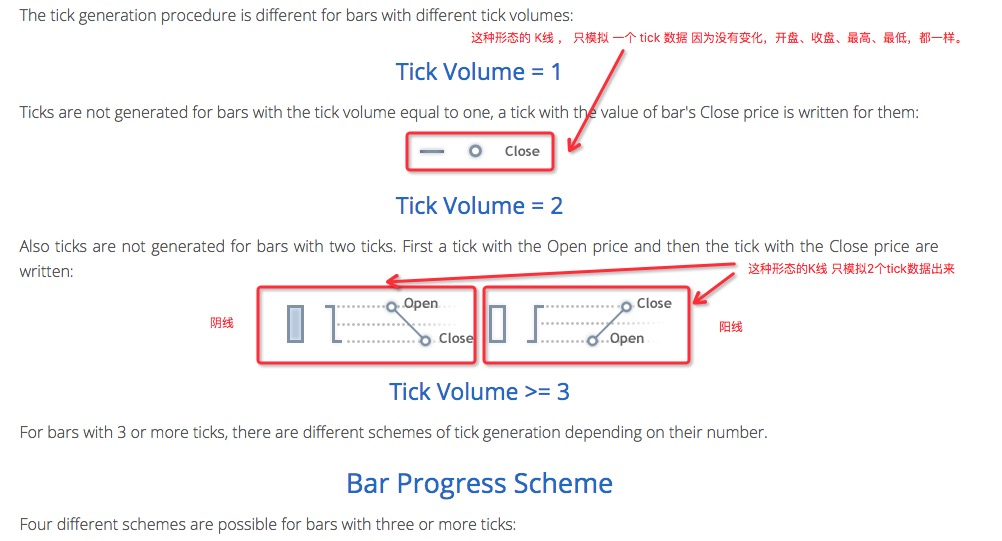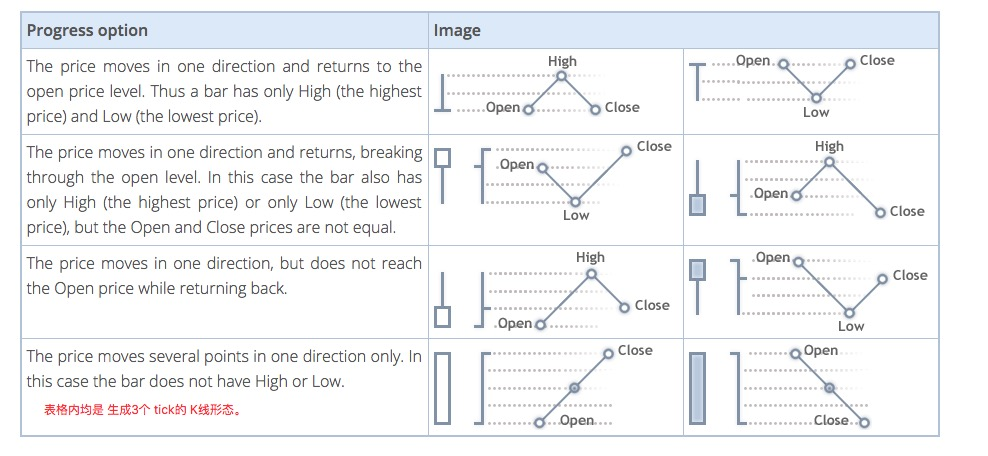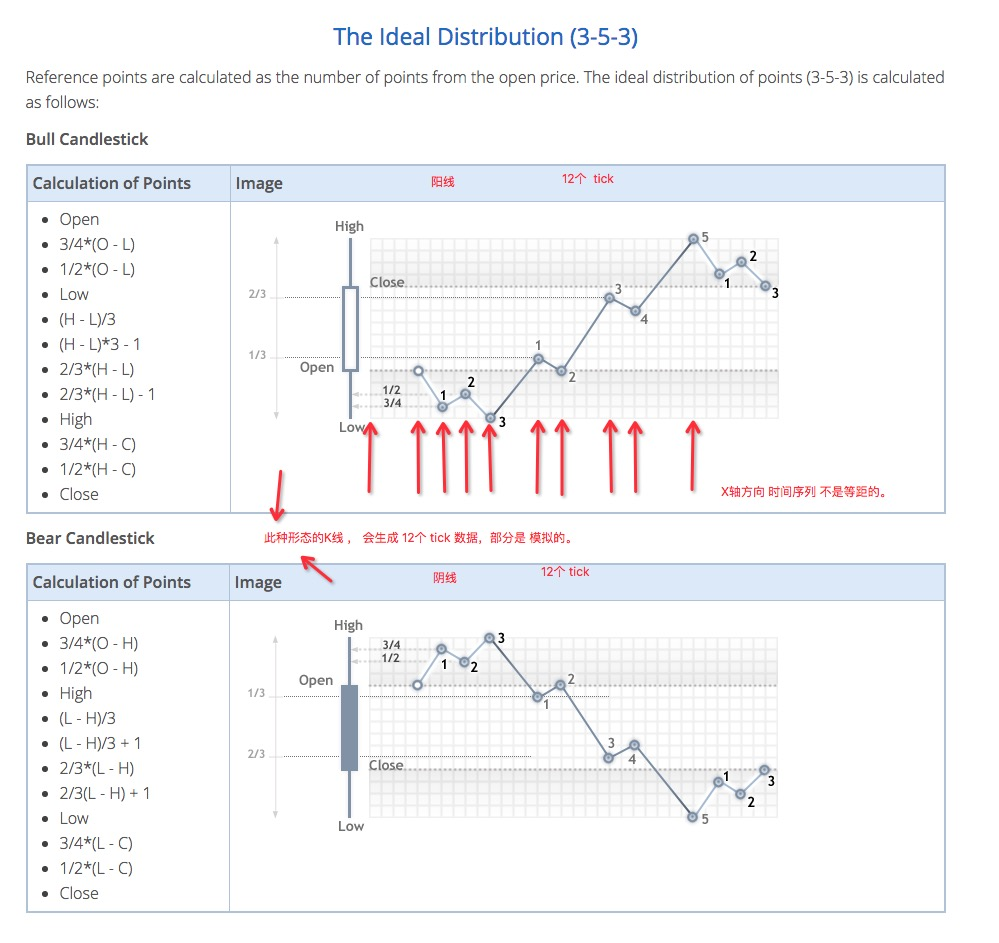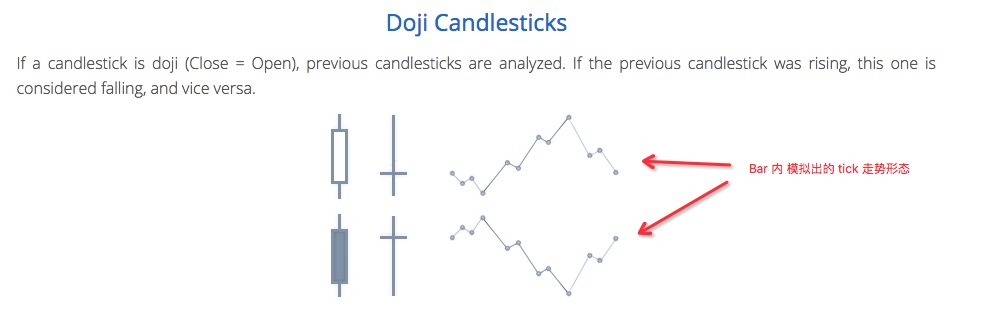发明者量化模拟级别回测机制说明

Author: 小小梦, Created: 2017-02-07 13:04:57, Updated: 2021-11-05 15:02:39

• 1、回测架构

发明者量化的回测中策略程序是完整的控制流程，程序是在按照一定的频率不停的轮询。各个行情、交易 API 返回的数据也是按照调用时刻，模拟实际运行时的情况。属于onTick级别，并非其它回测系统的onBar级别。更好的支持了基于Ticker数据的策略的回测（操作频率较高的策略）。

• 2、模拟级别回测和实盘级别回测的区别

• 模拟级别回测

模拟级别回测是按照回测系统的底层K线数据，按照一定算法在给定的底层K线Bar的最高价、最低价、开盘价、收盘价的数值构成的框架内，模拟出ticker数据插值到这个Bar的时间序列中。

• 实盘级别回测

实盘级别回测是真实的ticker级别数据在Bar的时间序列中。对于基于ticker级别数据的策略来说，使用实盘级别回测更贴近真实。 实盘级别回测，ticker是真实记录的数据，并非模拟生成。

• 3、模拟级别回测机制–底层K线

实盘级别回测没有底层K线选项（因为ticker数据都是真实的，不用底层K线来模拟生成）。 模拟级别回测中，基于K线数据模拟生成的ticker。这个K线数据就是底层K线。在实际使用模拟级别回测中，底层K线周期必须小于策略运行时调用API获取K线的周期。否则，由于底层K线周期较大，生成的ticker数量不足，调用API获取指定周期的K线时，数据会有失真的情况。在使用大周期K线回测时，可以适当调大底层K线周期。

• 4、底层K线如何生成ticker 数据

底层K线生成模拟ticker的机制和MT4是一样的： 相关链接• 5、生成ticker数据的算法代码

把底层K线数据模拟出tick数据的具体算法：

function recordsToTicks(period, num_digits, records) {
if (records.length == 0) {
return []
}
var ticks = []
var steps = [0, 2, 4, 6, 10, 12, 16, 18, 23, 25, 27, 29]
var pown = Math.pow(10, num_digits)

function pushTick(t, price, vol) {
ticks.push([Math.floor(t), Math.floor(price * pown) / pown, vol])
}

for (var i = 0; i < records.length; i++) {
var T = records[i]
var O = records[i]
var H = records[i]
var L = records[i]
var C = records[i]
var V = records[i]
if (V > 1) {
V = V - 1
}
if ((O == H) && (L == C) && (H == L)) {
pushTick(T, O, V)
} else if (((O == H) && (L == C)) || ((O == L) && (H == C))) {
pushTick(T, O, V)
} else if ((O == C) && ((O == L) || (O == H))) {
pushTick(T, O, V / 2)
pushTick(T + (period / 2), (O == L ? H : L), V / 2)
} else if ((C == H) || (C == L)) {
pushTick(T, O, V / 2)
pushTick(T + (period * 0.382), (C == L ? H : L), V / 2)
} else if ((O == H) || (O == L)) {
pushTick(T, O, V / 2)
pushTick(T + (period * 0.618), (O == L ? H : L), V / 2)
} else {
var dots = []
var amount = V / 11
pushTick(T, O, amount)
if (C > O) {
dots = [
O - (O - L) * 0.75,
O - (O - L) * 0.5,
L,
L + (H - L) / 3.0,
L + (H - L) * (4 / 15.0),
H - (H - L) / 3.0,
H - (H - L) * (6 / 15.0),
H,
H - (H - C) * 0.75,
H - (H - C) * 0.5,
]
} else {
dots = [
O + (H - O) * 0.75,
O + (H - O) * 0.5,
H,
H - (H - L) / 3.0,
H - (H - L) * (4 / 15.0),
H - (H - L) * (2 / 3.0),
H - (H - L) * (9 / 15.0),
L,
L + (C - L) * 0.75,
L + (C - L) * 0.5,
]
}
for (var j = 0; j < dots.length; j++) {
pushTick(T + period * (steps[j + 1] / 30.0), dots[j], amount)
}
}
pushTick(T + (period * 0.98), C, 1)
}
return ticks
}

More

psNOehs 收盘价不可能小于0的，判断C大小的else语句是不是有些多余？

FangBei 模拟回测的周期里面，1小时之后就直接是1天了，为什么没有2小时，4小时，6小时，12小时，这些常用的周期？

linsilence 不是0，是O，开盘价

bigbirdu 参考近期石油期货负价格事件，发现你真是细心啊

zrcahyx 模拟盘的话，底层k线周期对策略影响非常大，请问什么样的底层k线周期更解决实盘呢

xingbuxing okcoin一天的k线还是比较好弄的。关键分钟和逐笔的

xingbuxing 你有btc之前的历史数据吗？